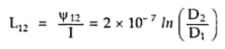### Inductance of a Conductor due to External Flux

Now we shall estimate the flux linkages of the conductor due to the external flux. For this we will consider the flux linkages of an isolated conductor due to that portion of the external flux which lies between two points distant and meters from centre of conductor P1 and P2 are two such points as shown in the Fig. 1.Fig. 1
The conductor shown in the Fig. 1 carries current I. The flux paths are concentric circles around the conductor between P1 and P2.
Consider a tubular element which is x meters from centre of conductor. The field intensity at this point is  Hx. The mmf around the element is
The flux density Bx at this point is given by
The flux dΦ in the tubular element is given by,
dΦ = Bx x dx x 1m (Axial length is considered as 1m)
The flux linkages dψ per meter equal to dΦ since flux external to the conductor links all the current in the conductor. The total flux linkage between P1 and P2 are obtained by integrating dψ from D1 to D
Since the    μ = μo μr
For Relatively permeability, μr = 1
The inductance due to flux included between P1 and P2 only is,In the external flux is considered to be extended from the surface of conductor to infinity then total flux linkages is given by,
Overall flux linkages is given by

#### 1 comment:

1.Very elaborated explanation. Very good!!